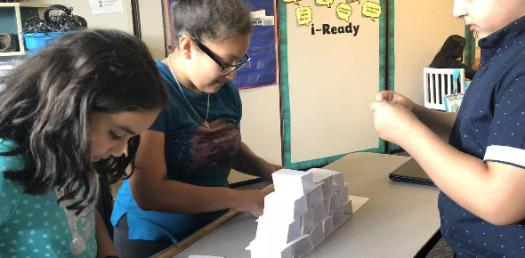15 Questions | Total Attempts: 463Settings• 1.
How many pounds are in 64 ounces?
• A.

8 Pounds

• B.

5 Pounds

• C.

6.4 Pounds

• D.

4 Pounds

• 2.
What is 85% of 30?
• A.

2.55

• B.

0.255

• C.

25.5

• D.

0.0255

• 3.
What is the surface area of a cube that has edges that are 2.5 centimeters long each?
• A.

6.25 Cubic Centimeters

• B.

15.625 Square Centimeters

• C.

18.75 Square Centimeters

• D.

37.5 Square Centimeters

• 4.
What value of x makes the statement "1/2 = x/10" true?
• A.

3

• B.

2

• C.

5

• D.

20

• 5.
What is the area of a square with sides that are each 3 feet long?
• A.

3 Square Feet

• B.

12 Square Feet

• C.

6 Square Feet

• D.

9 Square Feet

• 6.
How do you write 87% as a fraction in simplest form?
• A.

87/100

• B.

7-Aug

• C.

87/99

• D.

29/33

• 7.
What is the area of a circle with a radius of 6 inches if using 3.14 for pi?
• A.

28.26 Square Inches

• B.

113.04 Square Inches

• C.

226.08 Square Inches

• D.

37.68 Square Inches

• 8.
If Marc ran 4000 feet, what fraction of a mile, in lowest terms, did he run?
• A.

25/33 Miles

• B.

500/660 Miles

• C.

4000/5280 Miles

• D.

1000/1320 Miles

• 9.
What is the least common denominator of 3/4 and 1/5?
• A.

4

• B.

10

• C.

5

• D.

20

• 10.
What is the slope of the line y = 3x - 1?
• A.

3-Jan

• B.

1

• C.

3

• D.

-3

• 11.
What is the area of a triangle that has a base of 7 inches and a height of 5 inches?
• A.

35 Square Inches

• B.

18 Square Inches

• C.

70 Square Inches

• D.

17.5 Square Inches

• 12.
How many degrees are in one-half of a circle?
• A.

45 Degrees

• B.

90 Degrees

• C.

360 Degrees

• D.

180 Degrees

• 13.
How long is the hypotenuse of a right triangle if the legs are 6 inches and 8 inches in length?
• A.

14 Inches

• B.

9 Inches

• C.

10 Inches

• D.

100 Inches

• 14.
What is the slope of y = -2x + 7?
• A.

-2

• B.

2

• C.

7

• D.

-7

• 15.
How much time passes from 4:10 PM to 5:35 PM?
• A.

2 Hours 25 Minutes

• B.

2 Hours 15 Minutes

• C.

1 Hour 25 Minutes

• D.

1 Hour 35 Minutes

Related TopicsBack to top